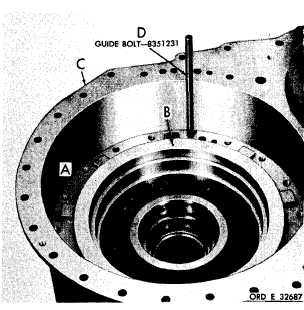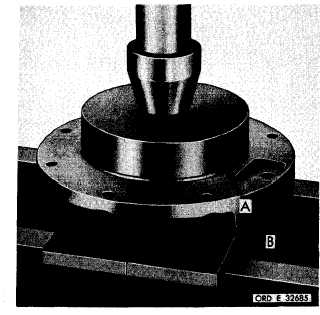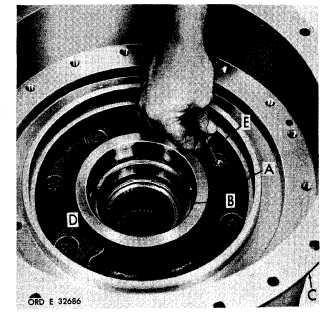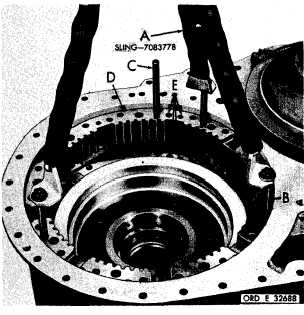Custom SearchC H A P    5,    SEC   X X X I II A S S E M B L Y P A R    7 ,     S T E P S    2  7 - 3 0 Figure   266   (Step   27) P r e s s    l e f t - o u t p u t    c l u t c h    s u p p o r t    b e a r i n g    ( A ) into   support   (B). Figure   267   (Step   28) Install   support   (A)   and   bearing   (B)   into   trans- mission   housing   (C).   Secure   sleeve   with   one 3 / 8 - 1 6    x    2    ( D )    a n d    s e v e n    3 / 8 - 1 6    x    1 - 1 / 4    ( E) self-locking    bolts.    Using    a    9/16-inch    wrench, torque   bolts   to   36-43   pound-feet. Figure   268   (Step   29) I n s t a l l    s t e e r    c l u t c h    p i s t o n    a s s e m b l y    ( A ) ,    l o - cating   oil   hole   (B)   in   the   assembly   in   relation t o    t r a n s m i s s i o n    h o u s i n g    ( C ) ,    a s    s h o w n .    I n- stall   guide   bolt   (D). Figure   269   (Step   30) U s i n g    s l i n g    ( A ) ,    t h r e e    7 / 1 6 - 1 4    x    5    b o l t s    ( B) and   guide   bolt   (C),   install   steer   clutch   anchor ( D ) .    I n d e x    h o l e s    ( E )    o f    t h e    a n c h o r    w i t h    t he holes   in   the   transmission   housing. 1 7 0